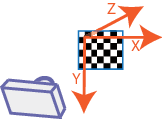# triangulate

3-D locations of undistorted matching points in stereo images

## Syntax

``worldPoints = triangulate(matchedPoints1,matchedPoints2,stereoParams)``
``worldPoints = triangulate(matchedPoints1,matchedPoints2,camProjection1,camProjection2)``
``````[worldPoints,reprojectionErrors] = triangulate(___)``````
``````[worldPoints,reprojectionErrors,validIndex] = triangulate(___)``````

## Description

example

````worldPoints = triangulate(matchedPoints1,matchedPoints2,stereoParams)` returns the 3-D locations of matching pairs of undistorted image points from two stereo images.```
````worldPoints = triangulate(matchedPoints1,matchedPoints2,camProjection1,camProjection2)` returns the 3-D locations of the matching pairs in a world coordinate system. These locations are defined by camera projection matrices. ```
``````[worldPoints,reprojectionErrors] = triangulate(___)``` additionally returns reprojection errors for the world points using any of the input arguments from previous syntaxes.```
``````[worldPoints,reprojectionErrors,validIndex] = triangulate(___)``` additionally returns the indices of valid and invalid world points. Valid points are located in front of the cameras.```

## Examples

collapse all

`load('webcamsSceneReconstruction.mat');`

Read in the stereo pair of images.

```I1 = imread('sceneReconstructionLeft.jpg'); I2 = imread('sceneReconstructionRight.jpg');```

Undistort the images.

```I1 = undistortImage(I1,stereoParams.CameraParameters1); I2 = undistortImage(I2,stereoParams.CameraParameters2);```

Detect a face in both images.

```faceDetector = vision.CascadeObjectDetector; face1 = faceDetector(I1); face2 = faceDetector(I2);```

Find the center of the face.

```center1 = face1(1:2) + face1(3:4)/2; center2 = face2(1:2) + face2(3:4)/2;```

Compute the distance from camera 1 to the face.

```point3d = triangulate(center1, center2, stereoParams); distanceInMeters = norm(point3d)/1000;```

Display the detected face and distance.

```distanceAsString = sprintf('%0.2f meters', distanceInMeters); I1 = insertObjectAnnotation(I1,'rectangle',face1,distanceAsString,'FontSize',18); I2 = insertObjectAnnotation(I2,'rectangle',face2, distanceAsString,'FontSize',18); I1 = insertShape(I1,'filled-rectangle',face1); I2 = insertShape(I2,'filled-rectangle',face2); imshowpair(I1, I2, 'montage');```## Input Arguments

collapse all

Coordinates of corresponding points in image one, specified as an M-by-2 matrix of M number of [x y] coordinates, or as one of the point feature objects described in Point Feature Types.

Coordinates of corresponding points in image one, specified as an M-by-2 matrix of M number of [x y] coordinates, or as one of the point feature objects described in Point Feature Types.

Camera parameters for stereo system, specified as a `stereoParameters` object. The object contains the intrinsic, extrinsic, and lens distortion parameters of the stereo camera system. You can use the `estimateCameraParameters` function to estimate camera parameters and return a `stereoParameters` object.

When you pass a `stereoParameters` object to the function, the origin of the world coordinate system is located at the optical center of camera 1. The x-axis points to the right, the y-axis points down, and the z-axis points away from the camera.Projection matrix for camera 1, specified as a 3-by-4 matrix. The matrix maps a 3-D point in homogeneous coordinates onto the corresponding point in the image from the camera. This input describes the location and orientation of camera 1 in the world coordinate system. `camProjection1` must be a real and nonsparse numeric matrix. You can obtain the camera matrix using the `cameraProjection` function.Camera projection matrices, passed to the function, define the world coordinate system.

Projection matrix for camera 2, specified as a 3-by-4 matrix. The matrix maps a 3-D point in homogeneous coordinates onto the corresponding point in the image from the camera. This input describes the location and orientation of camera 2 in the world coordinate system. `camProjection2` must be a real and nonsparse numeric matrix. You can obtain the camera matrix using the `cameraProjection` function.Camera projection matrices, passed to the function, define the world coordinate system.

## Output Arguments

collapse all

3-D locations of matching pairs of undistorted image points, returned as an M-by-3 matrix. The matrix contains M number of [x y z] locations of matching pairs of undistorted image points from two stereo images.

When you specify the camera geometry using `stereoParams`, the world point coordinates are relative to the optical center of camera 1.

When you specify the camera geometry using `camProjection1` and `camProjection2`, the world point coordinates are defined by the camera matrices.

The function returns `worldPoints` as data type `double` when `matchedPoints1` and `matchedPoints2` are of data type `double`. Otherwise, the function returns `worldPoints` as data type `single`.

Data Types: `single` | `double`

Reprojection errors, returned as an M-by-1 vector. The function projects each world point back into both images. Then, in each image, the function calculates the reprojection error as the distance between the detected and the reprojected point. The `reprojectionErrors` vector contains the average reprojection error for each world point.Validity of world points, returned as an M-by-1 logical vector. Valid points, denoted as a logical `1` (`true`), are located in front of the cameras. Invalid points, denoted as a logical `0` (`false`), are located behind the cameras.

The validity of a world point with respect to the position of a camera is determined by projecting the world point onto the image using the camera matrix and homogeneous coordinates. The world point is valid if the resulting scale factor is positive.

## Tips

The `triangulate` function does not account for lens distortion. You can undistort the images using the `undistortImage` function before detecting the points. Alternatively, you can undistort the points themselves using the `undistortPoints` function.

 Hartley, Richard, and Andrew Zisserman. Multiple View Geometry in Computer Vision. 2nd ed., 312-313. Cambridge, UK ; New York: Cambridge University Press, 2003.